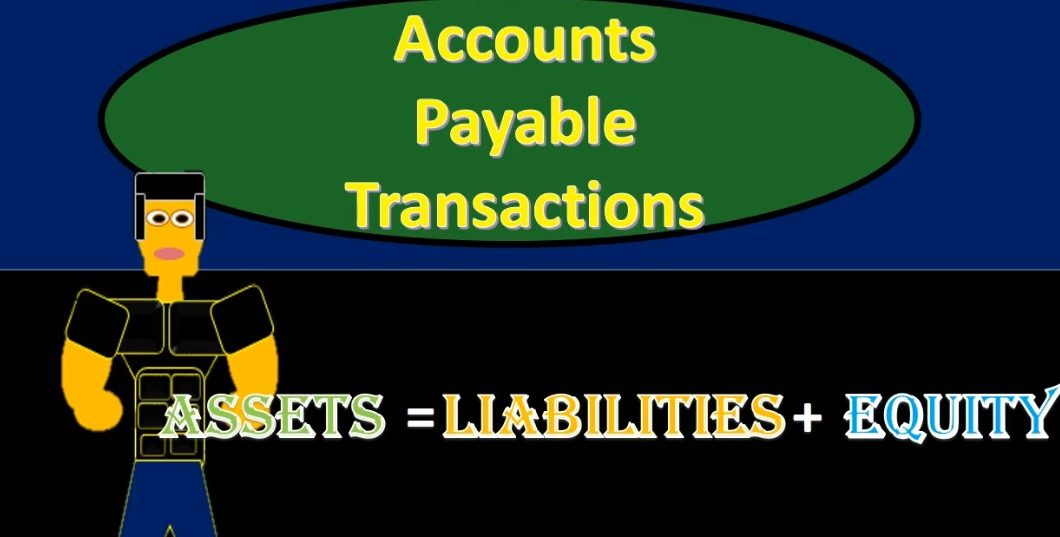## Accounts Payable Transactions Accounting Equation 170

So there’s gonna be problems later on where they’ll basically say, you know, you got to pay off something on account and you have to assume that the prior transaction took place. You got to kind of know in your mind how these things are related. So if we go through them by cycle that will help to achieve that goal. first transaction, we’re going to say purchase supplies on account. If we go through our list of questions, we’re going to say is cash affected? In this case? No, because we purchased it on account, then we’re going to ask what we’ve received, in this case supplies. So we got supplies, that is here, it’s going to be an asset. Therefore the asset is going to go up because we got more of them, then the only question is, what is the other account? It’s not a decrease to cash because we didn’t pay cash. And therefore we must be doing something somewhere else. That will be accounts payable, so accounts payable is going to increase by the same amount.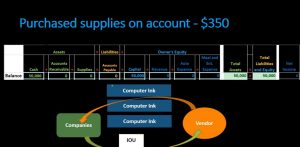03:47

How do we know that two ways. One, we know that supplies went up and if supplies went up on this side of the equal sign, then accounts payable must be going up on that side of the equal sign. That’s one way we know that the 350 is increasing. We also know that the accounts payable is kind of like something we don’t like. So the bad thing is going up, we owe more money after this transaction, therefore, accounts payable is increasing. Here is that transaction where we have this balance, beginning balance and what we actually did, meaning we increased the supplies and we are increasing the liabilities, that’s an increase to total assets and an increase to total liability. And that means that the equity then is going to remain the same. So within this transaction, then we see that assets are going to go up by that 315 liabilities going up by that 315. Therefore, the assets equal to liabilities and equity for that particular transaction. no impact on net income.

04:54

We didn’t need the total row meaning we’re going to add up what happened last time or what what the beginning balances were plus the current transaction will give us the total. For example, we had cash before plus zero is still the 50,000. The accounts receivable there was none, there are still none. The supplies were zero, we’re adding the 315 to get to 315. Accounts Payable was zero, we’re adding the 315 to get to 315. capital was 50,000, we’re adding zero to get to 50,000. revenue, auto meals all zero before nothing happened. All still zero after then if we were to add up the assets, liabilities and equity, assets have cash of 50,000 and supplies at 315 for a total of this 530 50,003 15 and the liabilities had 315 for accounts payable and capital 50,000 for a total of 50,000 15 no effect on net income.

06:03

Why? Because we purchased an asset. If we had purchased a an expense, or if we had consumed an expense, then it would be a decrease to net income. But when we just purchased the supplies, we have not yet consumed them in order to help us generate revenue, and therefore, it’s going to be an asset. Note that it has nothing to do with the fact that we bought it on account rather than paying cash as to why we are not expensing it. We’re not expensing it because we bought supplies an asset that has not yet been consumed, and we will expense it at the point in time it is consumed, not the point in time that we pay cash necessarily. Next transaction we’re going to say paid for purchase purchases in the past on account 315. So here are our beginning balance now. So our beginning balances are the same as we had in the prior slide. And now we’re going to say that we paid for something that we purchased in the past So first question is cash affected? We’re going to say yeah, we paid keyword being paid anytime it says paid, we can assume that cash is going down.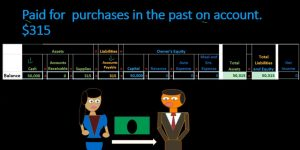07:10

And then we can just think about what the other account is. Now many times a problem will actually say, you know, we paid for supplies in the past or something like that, or we paid for supplies on account in the past, and they’ll actually give you the supplies and then we might think that we should be doing something to supplies. But of course, if it says on account, or if it says anything that we says we did it on in the past on account being a key term on account means either accounts receivable or accounts payable in this case accounts payable. If it is accounts payable, in this case, which it is, then we know it must be going down for at least two reasons. One, cash went down and cash is on this side or the left side of the equal sign. And if this is the other account affected on the right side of the equal sign, then it too Must be going down.

08:01

We also know that the receivable, the payable must be going down, because the payable represents what we owe what the business owes to a third party to a vendor. And this number represents we owe money. And we’re saying within this transaction that we pay paid money, we paid it off, and therefore the amount that we owe, it needs to be going down. So we can kind of double check ourselves and we should double check ourselves to see that this number is going down and kind of double check our entire transaction in that way. We see what happens then we’re going to say that cash is going down and we see that the liabilities are going down. So cash is decreasing here, we got the liabilities that decreasing on that side, we see that the total assets are going to equal total liabilities for this particular transaction.

08:55

Then we’re going to see the total here. total balance just bringing the balances down now Cash having 50,000 before we are subtracting the 3315 to bring it to 49,006 85. counts receivable was zero before still zero after supplies was 315. Before 315 plus zero is 315. We have accounts payable was 315. Before now we’re subtracting 315 to bring that balance down to zero, this is the typical kind of pattern we will see in accounts payable. It may not be just right next to each other where we you know, buy something on account and then pay off we might buy multiple things on on account and pay it off in some kind of installments. But we will typically see purchases and then payments and we should be able to match them up through the history of the account. We then have capital 50,000 plus zero 50,000 and revenue, expense and expense all zero before all zero after then If we were to add up our assets, our liabilities and equity, total assets are cash 49 685 and 315, totaling 50,000. And the liabilities and the equity now just being zero in the payable and 50,000 in capital to be a total of 50,000. no effect on net income, no effect so far on revenue or expenses.

10:03

Hello. In this presentation we will look at transactions related to the accounts payable cycle accounts payable transactions. We will record these transactions in accordance with the accounting equation that being assets equal liabilities plus equity. At the end of this we will be able to list transactions involving accounts payable and record transactions involving accounts payable using the accounting equation. We’re going to go through and list through the transactions, we’re going to look at our accounting equation, we’re going to have a beginning balance, list the transactions affected during one particular transaction, and then we’ll have an ending balance. In order to do this, we want to remember our accounting equation that’s been assets equal liabilities plus equity.

10:03

We’re going to then have our list of accounts within that area in that assets include cash, accounts receivable, supplies, liabilities deals with accounts payable, that is where we’ll we will be focusing most at this time, then the equity section is going to include the capital and the entire income statement, that being revenue and expenses. Note that the entire income statement is in this equity section. We currently have owner’s equity. But if it was a partnership, it would be partnerships equity, and if it was a corporation, it would be stockholders equity. The essence of equity as a total will be the same in that the entire income statement is going to be represented within equity when considering it through the accounting equation. We currently have total assets of 50,000 equaling total liabilities of 50,000 that being cash as the assets and capital as the liability on the equity and liabilities side.

10:03

There is no net income at this time. Net Income calculated as revenue minus expenses. Remember that we will be concentrated on the payable cycle. So this is going to be a cycle, we’re not looking at the transactions just by date here, but by cycle, which is often very practical, because one, that’s often the way we do it in practice, meaning you’re going to work we might work in a particular cycle, like the accounts payable cycle, and deal with the same types of transactions in order to specialize in some ways. And to it’s just easier to learn in cycles because of those processes being part of a set linked group of transactions. So you get to know the transactions that go together by doing this and by doing that, you can you can work through problems where you kind of have to assume what happened before.

10:28

Next transaction purchased auto service on account for 16. So we’re going to purchase Auto Service. First question is cash affected? We’re going to say no, because we purchased it on account, therefore, it’s going to be accounts payable that will be affected. I often think about what we receive as the second question in our thought process, what did we get? And that might not be as useful when we’re looking at the accounting equation. But it’s often more useful when we get to debits and credits I would get in the practice of saying what did we get and in this case, we got Auto Service. Now Auto Service isn’t buying the car, not an asset. Therefore, it’s going to be an expense over here in the equity section. Now expenses, we’re going to think they only go one way. Now typically when we think about debits and credits, they only go up, we can think they only go up here.

11:16

But we’re looking at this in relation to the equity section. So expenses are kind of like something we don’t like it’s bringing down total equity, you can think of total equity in terms of the income statement going the same way. As net income will go. So net income is calculated as revenue minus expenses. Therefore expenses only go up. As expenses go up, they bring down net income, and they bring down total equity. So when we’re considering an expense in terms of the equity section, we’re going to say it’s going to decrease the equity. Now I get I know that’s a little bit confusing when we’re thinking of expenses in terms of the accounting equation in terms of the equity section, but when we get to debits and credits, they’re always just going to be going in the debit direction. And you’ll hopefully get to know expenses pretty well, because we will be working with expenses a lot, there are typically more expenses than any other type of account.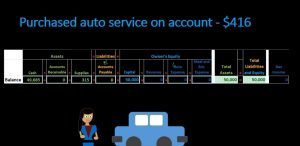12:13

So we’ll get a good feeling of what the expenses are doing. Therefore, the auto expense will be going down and we got to do something to some other accounts, we did not pay cash, it’s not going to be cash that it’s going down, it’s going to be the liability. Now we know the liability is going to be I’m sorry, the liability is going to be affected and it will be going up. Now we knew that this accounts payable this liability will be going up for a couple different reasons. One, if this side of the equal sign in auto expense is bringing down equity, equity and liabilities are on the same side of the equal sign. Then this account is the account that is affected which it is needs to be going up in order for the accounting equation to remain in balance.

12:59

We can also think through it in terms of the accounts payable at something we don’t really like the bad thing is going up, we bought something have not yet paid for it, we owe something in the future. That’s what the accounts payable represents. And that’s what’s happening here that the amount we owe is going up, we look at the transaction, and we’ve got 416 increase in the payable, and we have the auto expense. Again, it’s going up, you can think of expense going up, but it’s bringing down the equity section. So it’s bringing down net income, it’s bringing down equity. When you see a problem like this and a book problem, it may sometimes put minus signs here, so it’ll have it in the formula. Or they may ask you to put a minus sign when you enter the data. And this that’s just one of the problems again, with this accounting equation type of problem. When you move the debits and credits. It’s pretty it’s all straightforward.

13:49

That’s the point of debits and credits. But we’re trying to work with this accounting equation at first, just to get an idea of something that has pluses and minuses and it’s not a perfect thing. Because it does get confusing as we look through the areas over here with the plus and minus, so we’re subtracting the expense from total equity in this case, we see that there’s no effect on the accounting equation, because, well, there’s no effect on the total assets and the total liabilities and equity meaning assets are going to stay the same, liabilities are increasing, and capital is decreasing. So this side of the equal sign is remaining the same meaning liabilities and equity went up and down on meaning, accounts payable liability went up and expense brings equity down. So liabilities and expenses are equal to total assets in terms of what happened within this particular transaction. The liability increasing and the equity then decreasing.

14:53

There is an effect on net income, that being the expense net income calculated as revenue minus expenses. So if the revenue is going to net income is going down, note that the total equity will go in the same direction as net income. So when you times net income goes down, that’s going to bring total equity down as well. Assets then remain the same liabilities increase and equity decreases. If we look at the balance side then we’re going to say that cash started at 49 at 685. Nothing happened during this transaction ending balance 49 685 accounts receivable nothing before nothing after supplies started at 315 plus 03 15. Is the ending balance accounts payable was at zero before this, we are increasing it for 16 to 416, capital 50,000. Before this, nothing happened still 50,000 nothing in revenue, auto expense zero minus two for 16 to 416. Therefore If we add up the total assets, we have 49 685 plus 315, giving us 50,000. We add up the liabilities and equity for 16 plus the 50 minus the 416 will give us the 50,000. So we are in balance Vin, and net income is going down by the 416 in total as well, no revenue and only one expense of the 416.

16:29

Next transaction, purchase meals on account at 1009 50. Once again, is cash affected, we’re gonna say No, we didn’t. We didn’t pay cash, we purchased it on account. Therefore accounts payable is affected. I would typically get in the in the practice of working with meals and entertainment or the expense or what we got first, because this will be easier once we get to debits and credits. And that means we got meals and entertainment, meals and entertainment is an expense. It’s in the equity section. And it’s going to bring down total equity. So it’s going to be just like all other expenses, all expenses bring down equity. So we’re going to decrease the the meals and entertainment. And that means we must be doing something to some other account. It’s not going to be cash, we didn’t pay cash. So we’re not decreasing cash, we are increasing the bad thing rather than decreasing the good thing cash, we’re increasing the bad thing, accounts payable.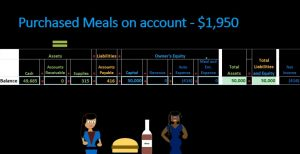17:24

So accounts payable will be the other side of this transaction, we know it’s going to increase for at least two reasons. One, we know that meals and entertainment is decreasing. It’s on the right hand side of this equal sign. And therefore this must be going up if it’s the other account affected, which it is in order to remain in balance. We also know that the bad thing is increasing in that accounts payable means we owe money. And it then is going up because we owe more money buying something on account that we have not yet paid for. If we look at that transaction, here it is. We have the accounts pay Apr increasing, we have the meals and entertainment, decreasing total equity. Again, it doesn’t mean that meals entertainment is going down, it means that meals and entertainment is decreasing total equity.

18:11

If we look at our equation, then we see that nothing happened to assets, liabilities are increasing and equity is decreasing from this transaction, we know that total assets then will be zero liabilities going up and liabilities and equity going down remains the total liabilities and equity will be zero. net income is affected here and that it is going down revenue minus expenses. We don’t have any revenue. We only have expenses for this time period so far. So we have a net loss in this case of 1950. We’re then going to bring down the balance so here is the balance we have 49 685 plus zero keeps us at 49 685. We had zero receivables and we end with zero In the receivables supplies at 315 plus 03 15 is what we end at there for 16. Accounts Payable plus 1009 50 gives us 2003 66.

19:13

We had capital 50,000 plus zero keeps us at 50,000. We have auto expense for 16 plus zero gives us 416. decrease in equity and meals entertainment zero plus or minus the 1009 50 keeps us at the 1009 50. If we are to add up then the total assets of 49 685 cash and 350 in supplies, we get to that 50,000 total assets. To add up liabilities and equity we get 2003 66 liabilities plus 50,000 Capital minus 416 in the auto expense minus meals and entertainment 1009 50 bringing us to 50,000 Net Income total effect as of the end of all the transactions we have done thus far 2006 366 that’s a loss, no income, no revenue yet, just expenses of 416. Auto and meals and entertainment of 1009 50. Next transaction paid for purchase made in the past is cash affected. We’re going to say yes, cash has been going down key term here paid anytime we see paid, we’re going to decrease cash, other side of the transaction, we bought something, but we don’t know exactly what it is.

20:38

So you might think it should be some type of expense or something. But we bought something that we purchased in the past, often it’ll say on account, and that means accounts payable in this case, meaning we’re paying off the liability. Now we know it’s going down for at least a couple reasons. One, we know that if this side of the equal sign is going down, then if this is the other account affected which it is, it must to be going down in order for the accounting equation to remain in balance. We also know what’s going down because accounts payable is a liability account. It represents us owing people money or the company owing people money.

21:14

And we have now paid that money, and therefore the amount owed should then go down. So here’s going to be the transaction, we’re going to decrease cash by the 416. We’re going to decrease accounts payable by 416. That means that total assets are decreasing by 416 with the cash and the accounts payable decreasing by 416. I’m sorry, that total liabilities and equity is also decreasing by 416. Due to the liabilities, no effect on net income here no effect on revenue and expenses. In other words, if we bring down the balance, we’re going to say, oh, let’s go through this. We’re going to say that the assets are going down liabilities are going down. The equity is remaining the same from this row from this transaction. Then we have the balances we’re going to say that the 49 685 minus the 416 brings us to 49 649 to 69. counts receivable zero plus zero is zero, supplies free 15 plus zero is 315. Accounts Payable 2003 66 minus 416 brings us to 1009 50. Capital 50,000 plus zero is 50,000. Revenue zero plus zero is zero. autorecover expense for 16, negative and zero is for 16 meals and entertainment 1009 50 zero brings us to 1009 50.

22:48

If we then add these up, we have the cash 49 to 69 plus zero plus 315 gives us 49 548 49. 84 and then the liabilities 1009 50 plus 250 thousand capital plus zero minus 416 minus 1009 50 brings us to a balance of liabilities and equity of 49,005 84 net income. That means we’re in balance here. Of course, net income then is actually a loss meaning revenue minus expenses of 416 expense plus the 1009 50 expense bringing us to the loss of 2003 66. If we look at the total transactions, then we can see the beginning balance the transaction brings us another balance plus the transaction brings us to another balance. So this is what the entire worksheet would kind of look like in a problem such as this. Notice it’s a kind of a messy worksheet when we see it laid out like this when we work at one thing at a time. It’s not too bad, but it’s a pretty messy worksheet when you see it all.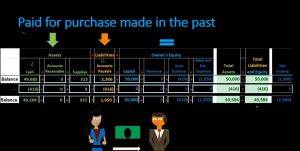24:00

At one point in time, it’s actually less messy when we move to debits and credits. So that’s why we move to debits and credits. Note here that we’re focusing in on the payables here. So the liabilities and the payables, we should see a pattern. In that we see that the we have the 315 happening here, and then 315 went out, increase in the balance, then decrease in the bounce back to zero, we have the 416 here, then the 416 went out. So we increase the 416 and then decrease the 416. And then we have the 1009 50, which never paid off, we still owe the 1009 50 at the end. That’s why that’s the ending balance here. That will be the typical pattern in a payable account. We’re going to increase it with a bill something that we incur we have not yet paid something that we incurred on account. And then at some point in the future, we will pay it and decrease that amount and we should then be able to match those two amounts out and match the increases match to the decreases as we go that should be the pattern that we will see. Back to the objectives. We are now able to list transactions involving accounts payable and record transactions involving record transactions involving accounts payable using the accounting equation.